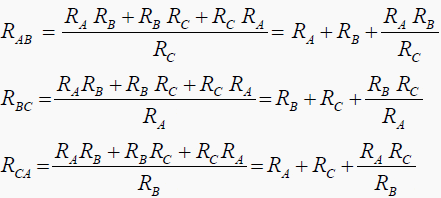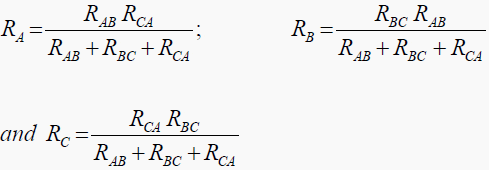Get access to premium HV/MV/LV technical articles, electrical engineering guides and papers. Check our new membership plans and prices!

# Elements of Electrical Engineering

## Star delta transformation

Concept of transformation: By the application of Kirchoffs Laws some problems cannot be solved and finds great difficulty due to number of equations. Such problems can be simplified by using star-delta or delta-star transformations.

The figure show two systems of connections of resistances. In star or ‘Y’ connection there is a common point for all the three resistors, and in delta or mesh connection the three are connected in series to form the loop and the junctions are takenout to form three supply points.

The common point in star connection is called ‘Neutral’. The delta connection will have no neutral point.

Assuming that the star connection is to be converted into delta connection. If the two networks are to be identical the resistance between any pair of lines will be the same when the third loop is opened.

### Tranformation form ( Y to Δ )#### How to remember?

The equivalent delta resistance between any two points is given by the sum of star resistances between those terminals plus the product of these two star resistances devided by the third resistor.

### Transformation from ( Δ to Y )#### How to remember?

As seen from the above expression it should be remembered that resistance of each line of the star is given by the product of resistances of the two delta sides that meet at its end divided by the sum of three delta resistance.

Get access to premium HV/MV/LV technical articles, electrical engineering guides, research studies and much more! It helps you to shape up your technical skills in your everyday life as an electrical engineer.

1.José Guillermo Medina Araujo
Apr 11, 2017

A quien corresponda
Es un excelente grupo, sera un honor participar con ustedes

Saludos gracias

2.Romualdo V. Ledesma
Sep 21, 2015

3.